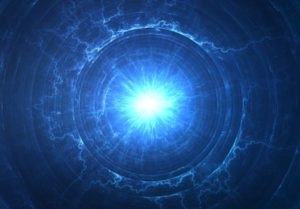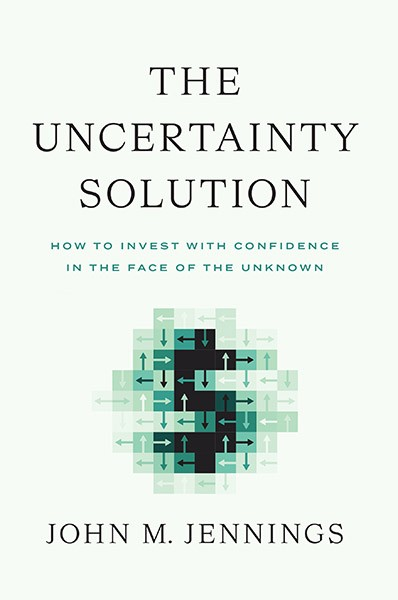## Zero-Point Field

by | Aug 4, 2017All around us everything is abuzz, so to speak.  Quantum particles do not sit still.  They move all over the place and jump around. In fact, virtual particles can spontaneously flash into existence from the energy of quantum fluctuations and then disappear.  Even in a vacuum there is a vast amount of energy due this so-called “zero-point energy” or it can be said that a “zero-point field” exists. The origin of the zero-point field is the Heisenberg Uncertainty Principle which was discussed in a previous IFOD.  That theory states that quantum particles, such as electrons, don’t exist in finite points in space, rather they exist within a realm of probability and when we try to detect their location we can determine either the particle’s location or its velocity, but not both.  Basically, what zero-point field reflects is an intrinsic quantum fuzziness in all energy and mass that exists at the quantum level.  It is all abuzz at the quantum level.

Thus, when you remove all energy from a system – what you are left with is the zero-point energy.  You cannot remove (and probably cannot make any use of) the zero-point energy – it is the lowest point.  But that being said, one topic that some scientists are working on is whether the energy in the zero-point field can be used.  There is a vast amount of energy trapped in the zero-point field and if that energy could somehow be harvested it could be used to power satellites or  interstellar space travel.  There are theoretical problems with doing this because quantum mechanics predicts that zero-point energy is the lowest amount of energy that can exist and if you could use it then you would have less than zero, so to speak.

What is really interesting about the zero-point field/energy is that it means that everything is connected.  Even in a vacuum objects are connected, in a sense, by the zero-point field.  Some connect great mystical or spiritual significance to this fact. If you search the world wide web for “zero point field” you’ll find a lot of scientific articles as well as a lot of far out speculation concerning the zero-point field.

In 1948 the  Dutch Physicist  Hendrik Casimir postulated that you could experimentally prove the existence of the zero-point field (which was merely a theory based on the mathematics of quantum mechanics).  His proposed experiment was to put two metal plates very close together (fractions of a millimeter) inside a vacuum and then measure whether there was a pressure pushing inward on the two plates.  The theory was that because the zero-point field has wave characteristics (according to the math) at small spaces, due to the wave-length of the zero-point field, there would be more zero-point pressure on the outside of the plates as opposed to the inside (not all the zero-point energy waves could fit between the plates).  This was a great idea, but in 1948 the technology did not exist to conduct the experiment.  The so-called “Casimir Effect” was not proven experimentally until 1997 when technology finally advanced to the point that experiments at University of Washington confirmed Dr. Casimir’s predicted pressure on the metal plates.  The Casimir Effect serves a useful point in illustrating that many times in physics and astronomy a mathematical theory may be valid, but not borne out by experiment for a long time.

This site uses Akismet to reduce spam. Learn how your comment data is processed.## View the Archives## Subscribe To The IFOD

Get the Interesting Fact of the Day delivered twice a week. Plus, sign up today and get Chapter 2 of John's book The Uncertainty Solution to not only Think Better, but Live Better. Don't miss a single post!